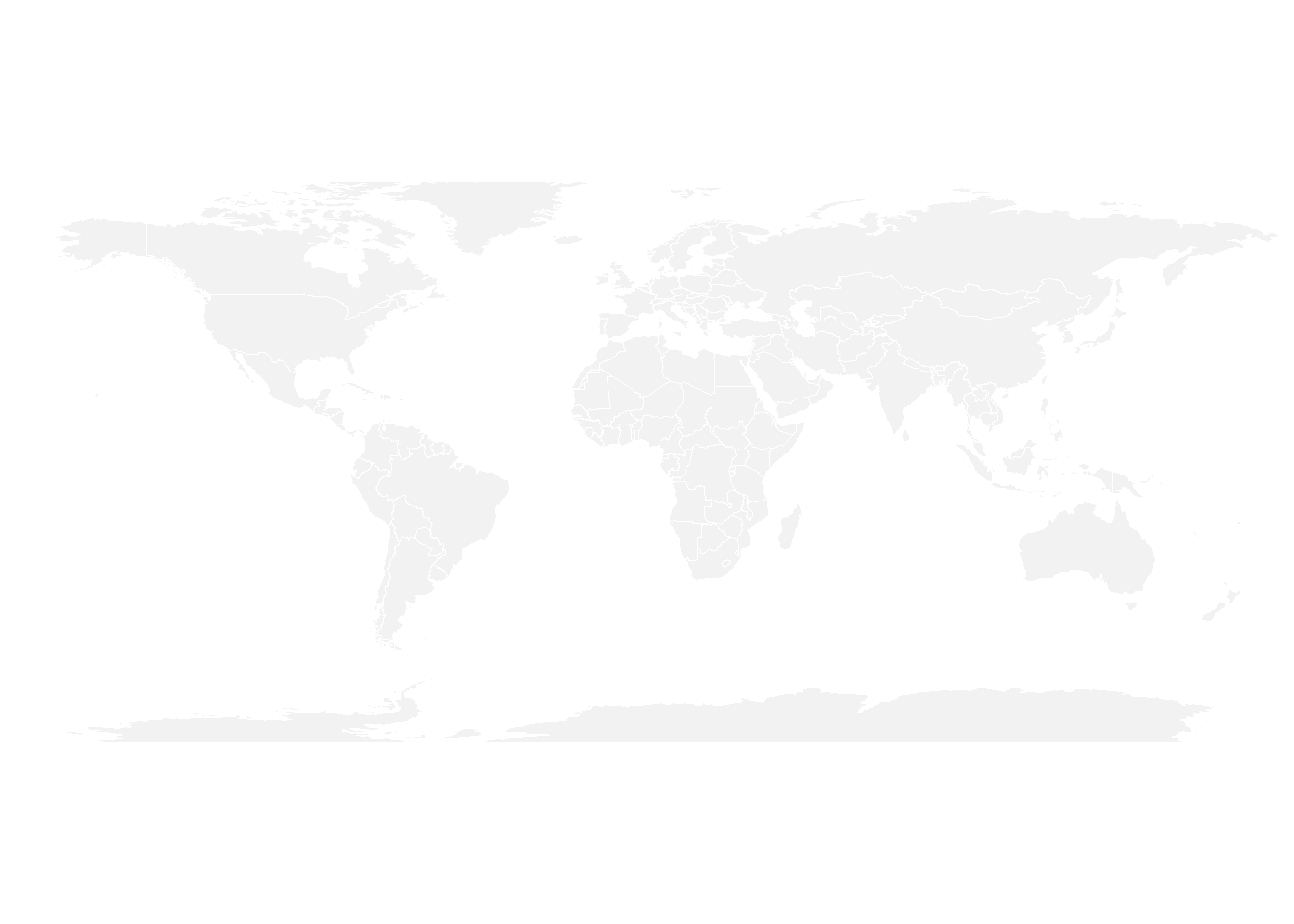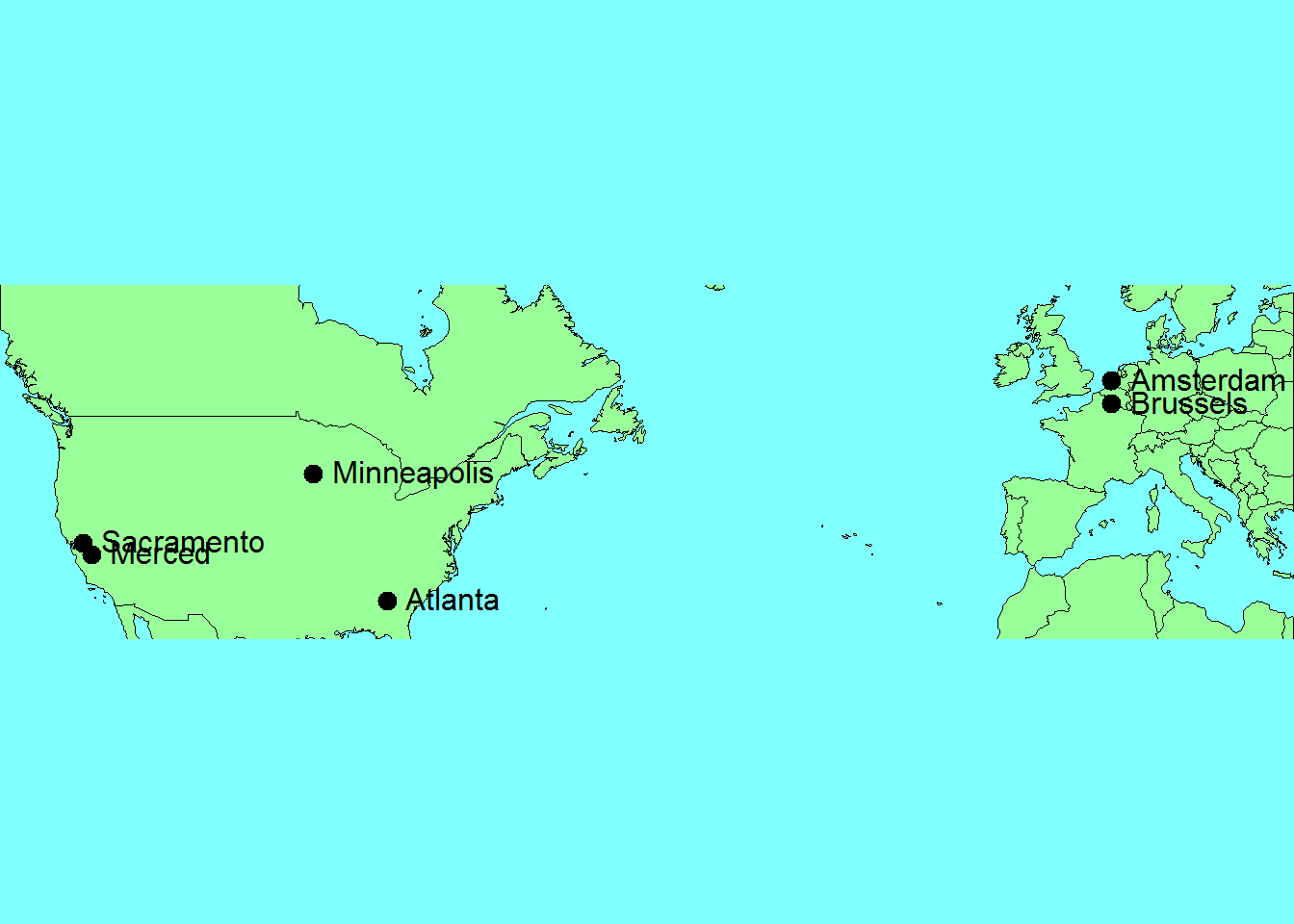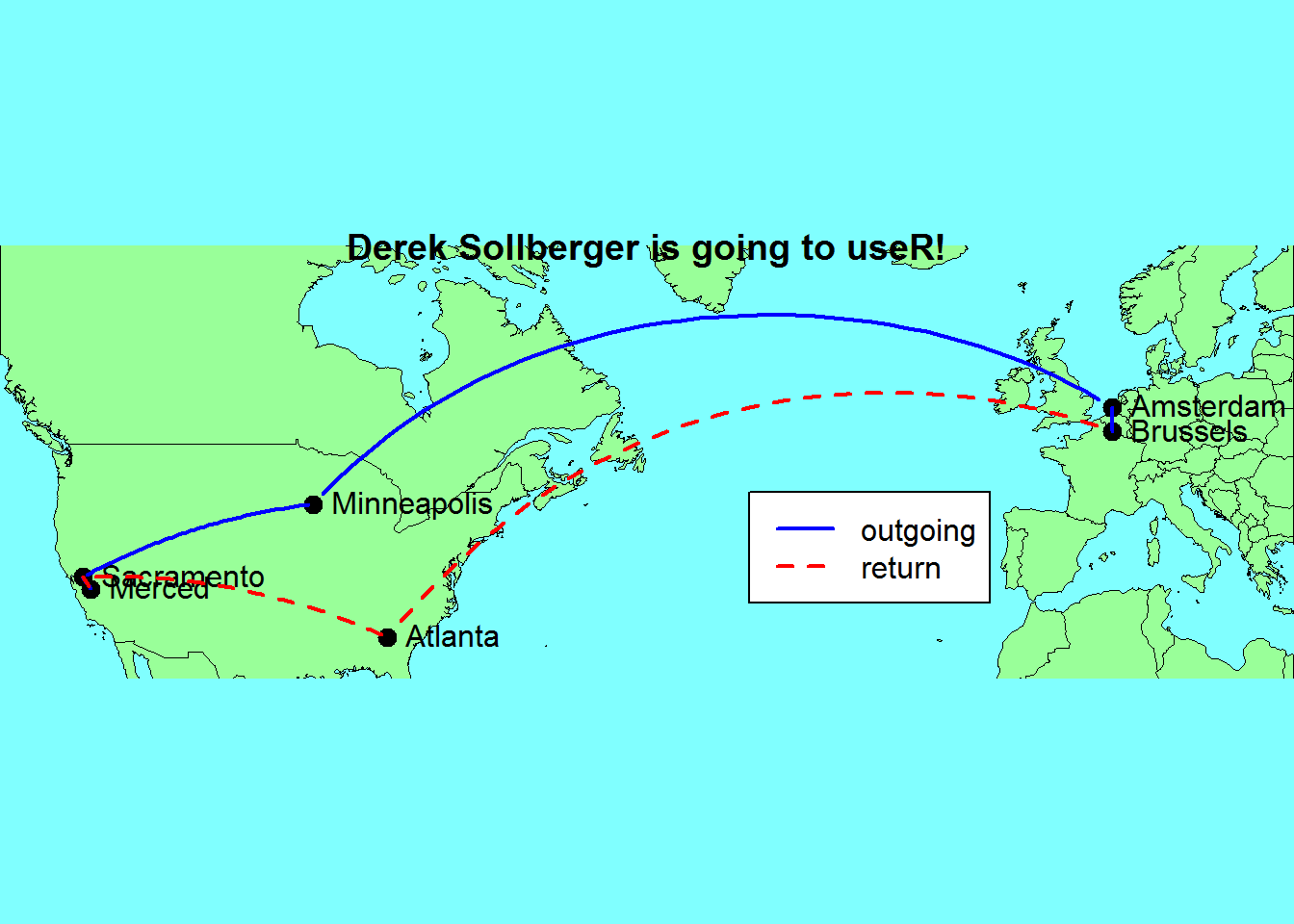Here I hope to draw a neat map for my itinerary to and from the upcoming useR conference in Brussels. I am inspired by and using the code from the post by Holtz on R Bloggers (https://www.r-bloggers.com/how-to-draw-connecting-routes-on-map-with-r-and-great-circles/).

library("geosphere")
library("maps")
library("tidyverse")

## Blank Slate

Note that there is a map function in both the maps and purrr packages, so I am going to be specific to use the maps version.

par(mar = c(0,0,0,0))
maps::map('world',col="#f2f2f2", fill=TRUE, bg="white", lwd=0.05, mar=rep(0,4),border=0, ylim=c(-80,80) )## My cities

Next, we need a data frame of longitude and latitude data for the cities.

myCities = as.data.frame(rbind(
Merced = c(-120, 37),
Sacramento = c(-121, 38),
Minneapolis = c(-93, 44),
Amsterdam = c(4, 52),
Brussels = c(4,50),
Atlanta = c(-84, 33)
))
colnames(myCities)=c("long","lat")

## Zooming In

I think we can use a subset of the world map for a better view. We can simply use the base package’s points and text functions to label the cities.

par(mar = c(0,0,0,0))
maps::map("world",
bg="#80ffff",
border=1,
col="#99ff99",
fill=TRUE,
lwd=0.05,
mar=rep(0,4),
xlim = c(-130, 25),
ylim = c(30, 60) )

points(x = myCities$long, y = myCities$lat,
cex = 2,
col = "black",
pch = 20)
text(rownames(myCities),
x = myCities$long, y = myCities$lat,
col = "black",
pos = 4)Now we will try out the gcIntermediate function in the geosphere package to draw the paths on the globe.

par(mar = c(0,0,0,0))
maps::map("world",
bg="#80ffff",
border=1,
col="#99ff99",
fill=TRUE,
lwd=0.05,
mar=rep(0,4),
xlim = c(-130, 25),
ylim = c(30, 65) )

points(x = myCities$long, y = myCities$lat,
cex = 2,
col = "black",
pch = 20)
text(rownames(myCities),
x = myCities$long, y = myCities$lat,
col = "black",
pos = 4)

# outgoing flight
lines(gcIntermediate(myCities[1,], myCities[2,]),
col = "blue", lwd = 2)
lines(gcIntermediate(myCities[2,], myCities[3,]),
col = "blue", lwd = 2)
lines(gcIntermediate(myCities[3,], myCities[4,]),
col = "blue", lwd = 2)
lines(gcIntermediate(myCities[4,], myCities[5,]),
col = "blue", lwd = 2)

# return flight
lines(gcIntermediate(myCities[5,], myCities[6,]),
col = "red", lty = 2, lwd = 2)
lines(gcIntermediate(myCities[6,], myCities[2,]),
col = "red", lty = 2, lwd = 2)
lines(gcIntermediate(myCities[2,], myCities[1,]),
col = "red", lty = 2, lwd = 2)

legend(-40,45,
c("outgoing", "return"),
col = c("blue", "red"),
lty = c(1,2),
lwd = c(2,2))
title("Derek Sollberger is going to useR!")